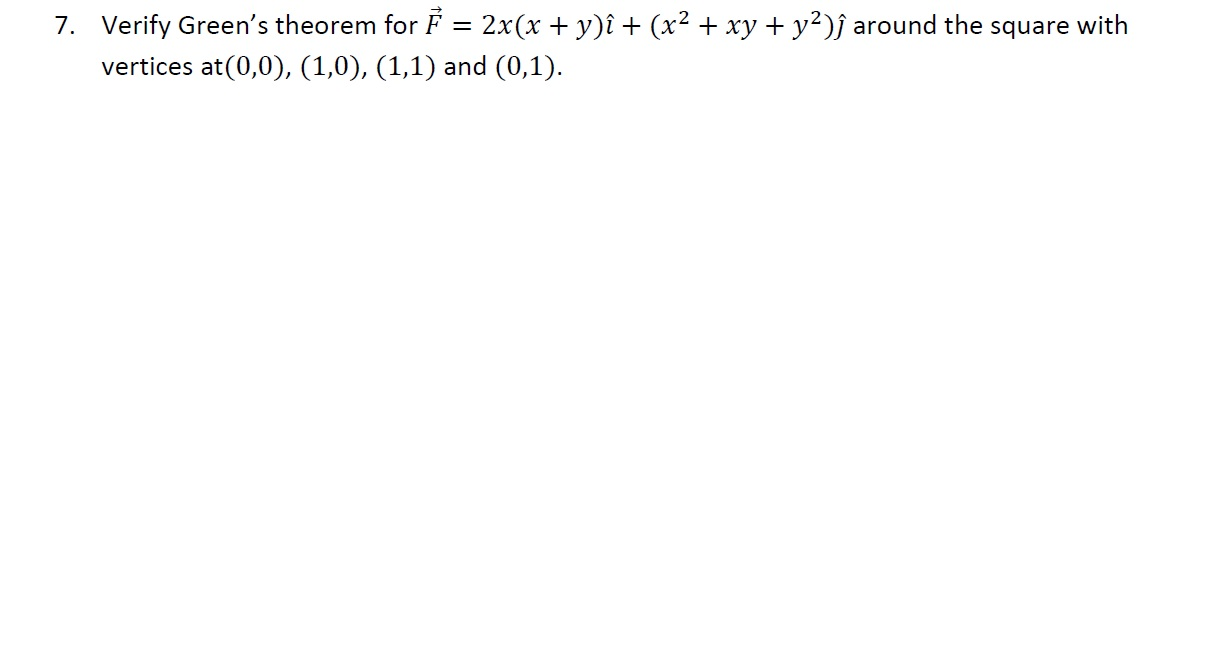Recent questions in VectorsConfused Fella 2022-05-15

### Find the area of the parallelogram with vertices A(0,1), B(3,0), C(5,-2), and D(2,-1).Lexi Chandler 2022-05-07 Answered

### (-2,3)+(-1,6)=?Regina Ewing 2022-05-07 Answered

### (-4,7)+(4,-4)=?quorums15lep 2022-05-06 Answered

### (-5,4)+(7,7)=?hovudverkocym6 2022-05-06 Answered

### What is (3,-4) plus (2,-4)?mikior9t3ue 2022-05-06 Answered

### (5,-7)+(4,5)=?kromo8hdcd 2022-05-05 Answered

### What is (-7,3) plus (4,4)?Dashawn Robbins 2022-04-22 Answered

### Given the two vectors, $\stackrel{\to }{A}=2\stackrel{^}{i}+4\stackrel{^}{j}$, and $\stackrel{\to }{B}=-1\stackrel{^}{i}+6\stackrel{^}{j}$, what is the angle between them in degrees?hisyhauttaq84w 2022-04-04 Answered

### What is (4,-1) plus (4,1)?adocidasiaqxm 2022-04-04 Answered

### (-6,-3)+(-6,-3)=?dumnealorjavgj 2022-04-04 Answered

### (-7,-1)+(-4,-1)=?agrejas0hxpx 2022-04-04 Answered

### What is (-6,4) plus (-6,-5)?brunocazelles4dvb 2022-03-25 Answered

2022-03-23

### Find the area of the triangle with vertices A(0,0,0), B(2,1,1), and C(−1,−1,−1), and find its angle at the vertex A.punniya.v 2022-01-19 Answered

### please provide answer for this questionpunniya.v 2022-01-19 Answered

### please provide answer for this question Show that the vector field by ⃗= (x^2+4yz)̂+ (y^2+4zx)̂+ (z^2+4xy) ̂ is irrotational. Hence find the scalar potential.eozoischgc 2022-01-07 Answered